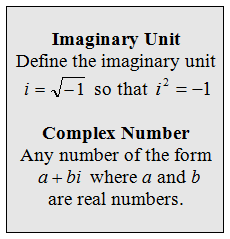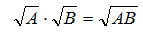## Pages

Showing posts with label complex numbers. Show all posts
Showing posts with label complex numbers. Show all posts

### Complex Numbers and Complex Solutions

Up to this point, we have been working exclusively with real numbers.  Now we shed this limitation and allow for a much broader range of problems.With these definitions, we have greatly expanded our space of numbers.  Notice that any real number is also a complex number, for example 5 = 5 + 0i.  Here the real part is 5 and the imaginary part is 0.  Next we consider powers of i.Instructional Video: Introduction to Complex Numbers

Simplify.Adding and subtracting complex numbers is just a matter of adding like terms. Be sure to use the order of operations and add real and imaginary parts separately.We have to use a bit of caution when multiplying complex numbers.  First, we will run into i^2 often. In this case, we will replace them all with -1.  In addition, the propertyis true only when either A or B is non-negative; so it is best to simplify using the imaginary unit before multiplying.Tip: Make use of the imaginary unit if the radicand is negative before trying to simplify.

Multiply.Multiplying complex numbers often requires the distributive property.Dividing complex numbers requires techniques similar to rationalizing the denominator.

Divide.
When a complex number is in the denominator, multiply numerator and denominator by its conjugate.
Sometimes quadratic equations have complex solutions.

Solve by extracting roots.
Solve by completing the square.
Solve using the quadratic formula.
If when solving for the x-intercepts, the solutions come out complex then there are none. This tells us that the parabola is completely above or below the x-axis.

Graph and label all important points:
Graph and label all important points:

Video Examples on YouTube: